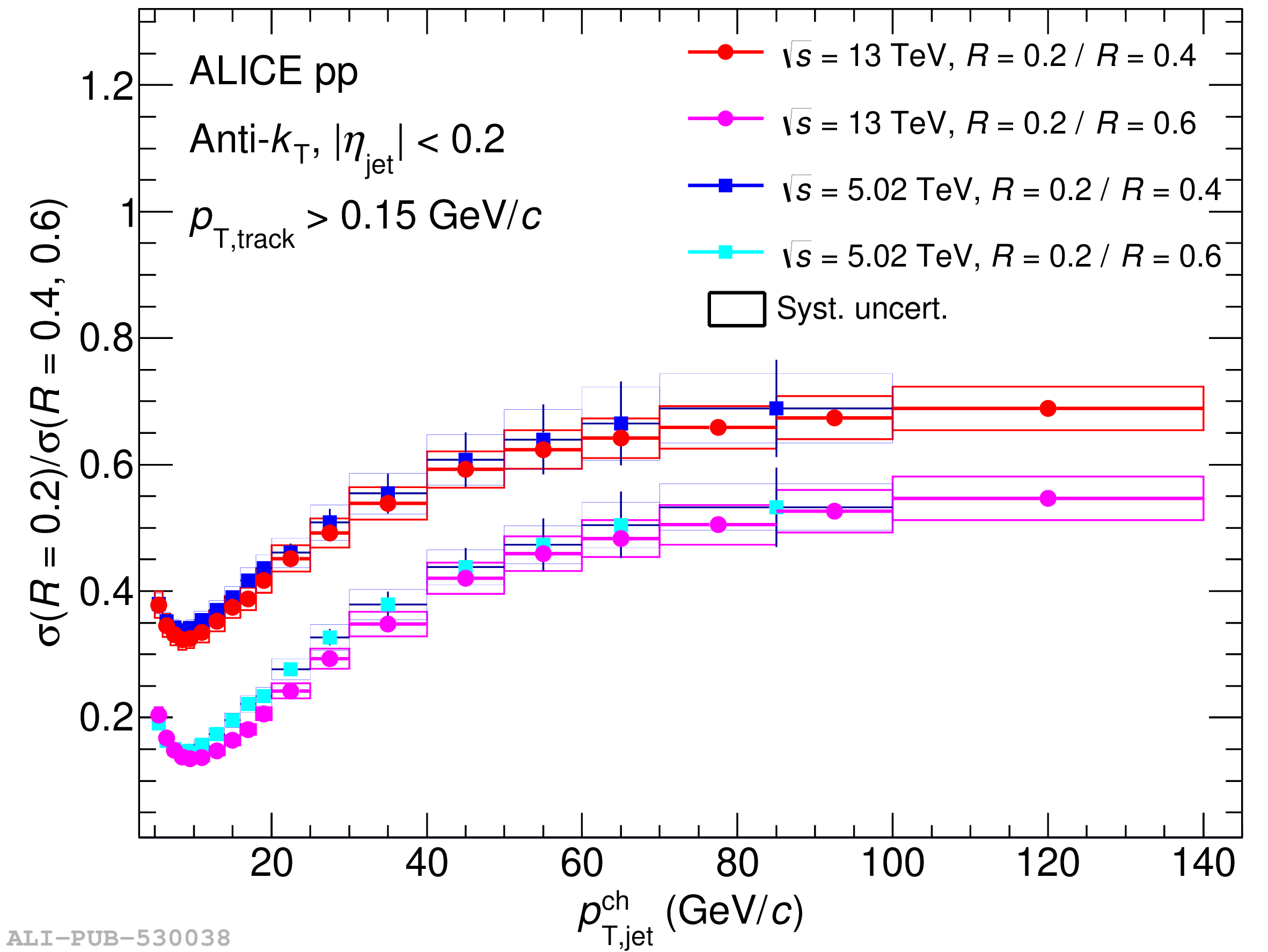# Figure A.4

 Comparison of charged-particle jet cross section ratios for $\sigma(R=0.2)/\sigma(R=0.4)$ and $\sigma(R=0.2)/\sigma(R=0.6)$ without UE subtraction in pp collisions at $\sqrt{s} = 13$ and $5.02$ $\mathrm{TeV}$ .